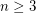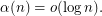Azarija, Jernej

Minimal graphs with a prescribed number of spanning trees ★★

Author(s): Azarija; Skrekovski

Conjecture   Letbe an integer and letdenote the least integersuch that there exists a simple graph onvertices having preciselyspanning trees. Then